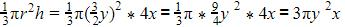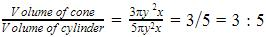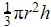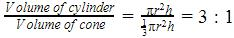Courses

# Test: Volume Combination Of Solids

## 20 Questions MCQ Test Mathematics (Maths) Class 10 | Test: Volume Combination Of Solids

Description
This mock test of Test: Volume Combination Of Solids for Class 10 helps you for every Class 10 entrance exam. This contains 20 Multiple Choice Questions for Class 10 Test: Volume Combination Of Solids (mcq) to study with solutions a complete question bank. The solved questions answers in this Test: Volume Combination Of Solids quiz give you a good mix of easy questions and tough questions. Class 10 students definitely take this Test: Volume Combination Of Solids exercise for a better result in the exam. You can find other Test: Volume Combination Of Solids extra questions, long questions & short questions for Class 10 on EduRev as well by searching above.
QUESTION: 1

### The radius of a cylindrical tank is 28m. If its capacity is equal to that of a rectangular tank of size 28mx16mx11m, then its depth is​

Solution:

Let d be the depth of the cylindrical tank. According to the given information, π(28)2 xd=28x16x11
⇒ d=2
Thus, the depth of the cylindrical tank is 2 m.

QUESTION: 2

Solution:
QUESTION: 3

### A cone and a cylinder have their heights in the ratio 4: 5 and their diameters are in the ratio 3: 2. The ratio of their volumes will be​

Solution:

Height of cone =4x
Height of cylinder=5x
Diameter of cone=3y
Diameter of cylinder=2y
Volume of cone=Volume of cylinder= πr2h=y2*5xQUESTION: 4

Volume of a hollow right circular cylinder is​

Solution:
QUESTION: 5

A container is in the form of a hemispherical bowl mounted by a hollow cylinder. The diameter of the sphere is 24 cm and the total height of the container is 16 cm. Its capacity is​

Solution:
QUESTION: 6

The volume of a sphere (in cu. cm) is equal to its surface area (in sq. cm). The diameter of the sphere (in cm) is

Solution:
QUESTION: 7

A solid toy is in the form of a right circular cylinder with a hemispherical shape at one end and a cone at the other end. Their common diameter is 4.2 cm and the heights of the cylindrical and conical portions are 12 cm and 7 cm respectively. The approximate volume of the toy will be​

Solution:
QUESTION: 8

A cylinder, a cone and a hemisphere are of equal base and have the same height. The ratio of their volumes is​

Solution:
QUESTION: 9

A spherical steel ball is melted to make 8 new identical balls. Then the radius of each new ball is how much times the radius of the original ball​

Solution:
QUESTION: 10

A river 3 m deep and 10 m wide is flowing at the rate of 2 km per hour. How much water will fall into the sea in a minute?​

Solution:
QUESTION: 11

A largest sphere is carved out of a cube of side 7cm. The volume of the sphere is​

Solution:
QUESTION: 12

A cylinder whose height is two thirds of its diameter has the same volume as a sphere of radius 4 cm. The radius of the base of the cylinder will be​

Solution:
QUESTION: 13

The radii of two right circular cylinders are in the ratio 2: 3 and their heights are in the ratio 5: 4. The ratio of their volumes will be

Solution:
QUESTION: 14

A cylindrical tub of radius 5 cm and length 9.8 cm is full of water. A solid in the form of a right circular cone mounted on a hemisphere is immersed in the tub. If the radius of the a hemisphere is 3.5 cm and height of cone outside the hemisphere is 5 cm, find the volume of the water left in the tub.​

Solution:
QUESTION: 15

The surface area of a cube is equal to the surface area of a sphere. The ratio of their volumes will be

Solution:
QUESTION: 16

The volume of a largest sphere than can be cut from cylindrical log of wood of base radius 1 m and height 4 m is:

Solution:
QUESTION: 17

A cylindrical vessel of diameter 42 cm and height 40 cm contains water up to a depth of 16 cm. Now a solid iron cylinder, with diameter 14 cm and height 32 cm is placed upright in the cylindrical vessel. The volume of the water required to just submerge the solid cylinder will be

Solution:
QUESTION: 18

A rectangular paper is 44 cm long and 20 cm wide. A cylinder is formed by rolling the paper along its length. The volume of the cylinder will be​

Solution:
QUESTION: 19

A cylinder, a cone and a hemisphere have equal base and height. Find the ratio of their volumes

Solution:
QUESTION: 20

A cylinder and a cone are of same base radius and of same height. The ratio of the volume of cylinder to that of the cone is:​

Solution:

We have radius of cylinder and cone = r
Height of cone and cylinder = h
Volume of cone =Volume of cylinder = πr2h